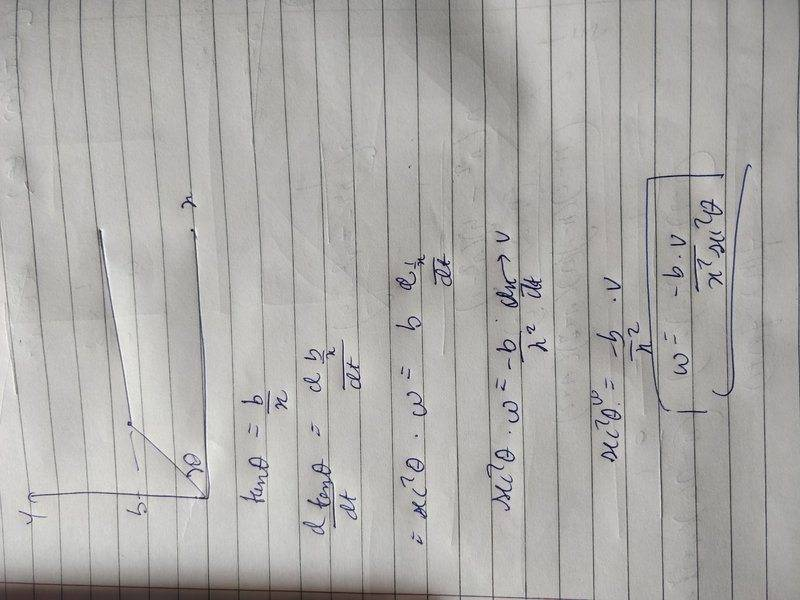# Circular motion

## Homework Statement

A particle is moving parallel to x-axis in the positive direction with velocity v such that at all the instants the y -axis component of its position vector is constant and is equal to 'b'. Find angular velocity about origin.

## The Attempt at a Solution

I thought that angular velocity would be the rate of change of the angle of the position vector of the particle with the origin, let's say theta. So I wrote tan (theta) = b/x and differentiated with time but got the wrong answer.

Delta2
Homework Helper
Gold Member
Seems to me your general approach is correct, so either you did a mistake in the differentiation or on the afterwards algebraic manipulation that is needed in order to find the angular velocity.

Please post your work with as much detail as you can so we ll be able to pinpoint your mistakes.

haruspex
Homework Helper
Gold Member
2020 Award
perhaps it is a matter of whether you take the angle as from the x axis or from the y axis.

Delta2
Homework Helper
Gold Member
The answer I got myself, taking the angle from the x-axis is ω=bv/(b2+x2). replace x=vt to get it as a function of time t. Is that the answer from your book, or is that what you calculated?

The answer I got myself, taking the angle from the x-axis is ω=bv/(b2+x2). replace x=vt to get it as a function of time t. Is that the answer from your book, or is that what you calculated?
Thats what I am getting, but the answer is different in the book, I checked the solutions and what they had done is that they took component of velocity perpendicular to position vector and then differentiated, I have a feeling that its wrong because thats not what my teacher taught, what do you think?#### Attachments

perhaps it is a matter of whether you take the angle as from the x axis or from the y axis.
No the book had a diagram, and angle is clearly marked with horizontal, similar to the one I have drawn in the post above.

Delta2
Homework Helper
Gold Member
I think the two approaches should give same result. Can you give us what is the final answer from your book?

I think the two approaches should give same result. Can you give us what is the final answer from your book?
Its [v sin^2(theta)]/b

haruspex
Homework Helper
Gold Member
2020 Award
Its [v sin^2(theta)]/b
are you quite sure that is different? Note that you were not given x, so you should eliminate that from your answer.

Delta2
Homework Helper
Gold Member
It is the same as we calculated, it is easy to see that ##\sin^2(\theta(t))=\frac{b^2}{b^2+x(t)^2}##

Ohh I didn't see that, thank you! But how do I justify the book's approach, I don't understand why their answer is correct. They have divided the magnitude of component of velocity perpendicular to position vector by the magnitude of position vector.

haruspex